# 1.- Introduction

The Great Pyramid of Giza, in Egypt, fascinates many of us. It is an astonishing construction built with an incredible accuracy, far beyond the one achievable by our current technology. In this article we discuss some important properties of its design which are closely related to Sacred Geometry. We will show that the ratio of the apotem to half the base obeys the Golden Ratio, and that the perimeter of its base equals that of a circle with a radius equal to its height. We will also see that the angles of the Great Pyramid hide the Euler number. As a preparing we need to review the properties of the Kepler triangle and the problem of the Squaring of the Circle. Then we will show that the dimensions of the Earth and Moon obey the same proportions as the dimensions of the Great Pyramid, and that they can be directly obtained using the Phythagorean 3-4-5 triangle. And finally we will show how the exact dimensions of the Great Pyramid obey a very simple formula related to the cubes of some specific integer values.# 2.- Squaring the Circle

Consider a general right-angle triangle of base b, height h and hypothenuse a. Its circumscribing circle will have the same perimeter as a square of size h provided that the sides of the triangle satisfy the relationship (Figure 1a)

$πa=4h⇒ah=4π$

This is often known as the Squaring of the Circle. If the square is formed with a side equal double the triangle base 2b, the same problem is usually stated in terms of the circle that has a radius equal the triangle height h (Figure 1b). In this case, the squaring of the circle is achieved if the height and the base are related as

$2πh=8b⇒hb=4π$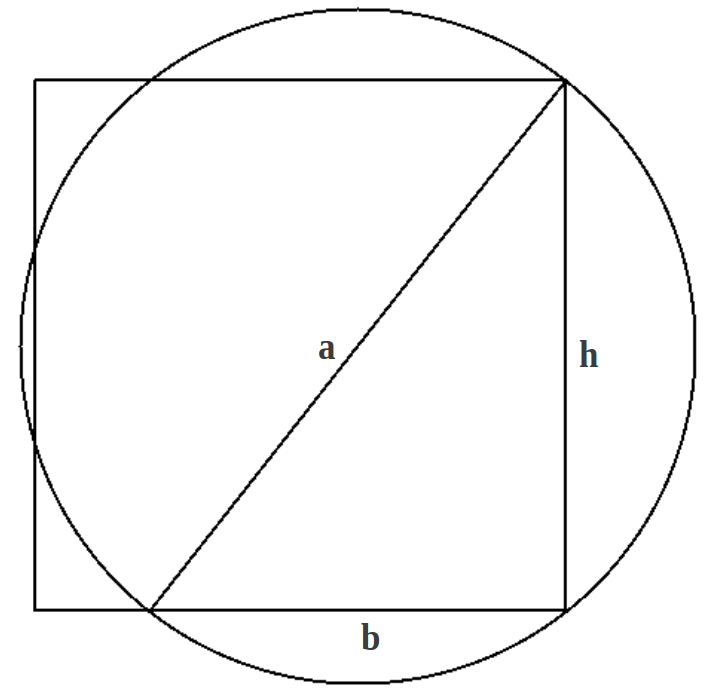(a) (b) Figure 1: Two ways of squaring the circle starting from the same right-angle triangle.

It is obvious that the two relationships above cannot be satisfied simultaneusly, because given two sides of a right-angle triangle the third one must obey Phythagoras theorem. However, if we relax the above equalities to good approximations, we see that the sides of the triangle would have to be related in a geometric progression:

$ah≈4πhb≈4π→h2=ab$

A question arises naturally: is that possible? And in this case, which is the accuracy of the squaring of the circle approximated that way?

# 3.- Kepler triangle

We know there is only one right-angle triangle whose sides are in an arithmetic progression, the Phythagorean 3-4-5 triangle. Similarly, there is only one right-angle triangle with edge lengths in a geometric progression, known as Kepler triangle. Do you guess what magic number is involved in this triangle? Yes, as it could not be another way, it is the Golden Ratio! The sides of Kepler triangle obey the progression $1:\sqrt{\phi }:\phi$. The reader can check that this triangle approximates the Squaring of the Circle with an error of 0.096% (Figure 2), which exemplifies the known relationship between π and φ:

$φ≈4π$Figure 2: The squaring of the circle in Kepler triangle.

In the following section we will show that the Great Pyramid squares the circle with a better approximation than the one obtained from Kepler triangle.

# 4.- Sacred Geometry in the Great Pyramid

The following figure shows the definition of the apotem (a), the height (h) and half base (h) of a typical square pyramid. At the right we list the accepted dimensions of the Great Pyramid in three different units of measurement:2b h 440 ec 280 ec 755.9 ft 481.0 ft 230.4 m 146.6 m Table 1: Accepted dimensions of the Great Pyramid of Giza (from Wikipedia), given in egyptian cubits (ec), in feet (ft) and in meters (m).

The first property that we want to highlight is the presence of the Golden Ratio in the Great Pyramid with a high accuracy, as the ratio of the apotem to half the base:

$ab=2202+2802220=1.618590347≡φr=φ±0.03%$

This ratio provides an approximation φr to the Golden Ratio φ. As a consequence, the triangle a-b-h shown in Table 1 is very close to a Kepler triangle. Therefore, the Great Pyramid also exemplifies in stone the Squaring of the Circle! If you draw a circle with radius equal to its height, its perimeter will be the same as the base of the pyramid with an error as low as 0.04%:

$square⟶8b=1760 eccircle⟶2πh=1759.2919 ec$

The fact that the Great Pyramid approximates the Squaring of the Circle with a better accuracy than Kepler triangle is due to the use of the rational approximation of π/2 in the ratio of height to base (Figure 3):

$π2≈117=440280$Therefore the rational approximation πr of π is given by:

$πr≡227=3.142857143=π±0.04%$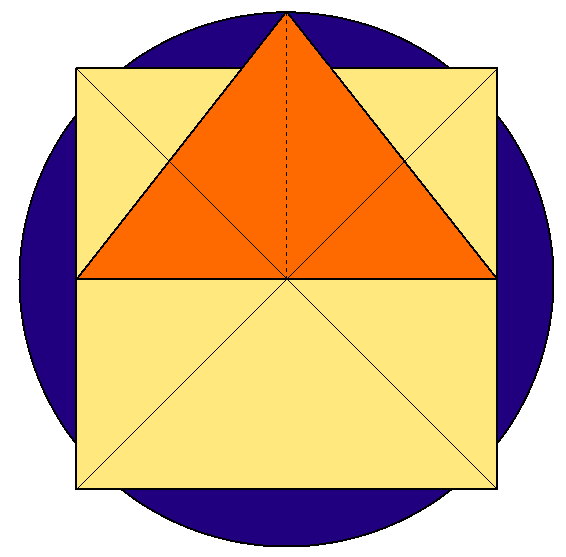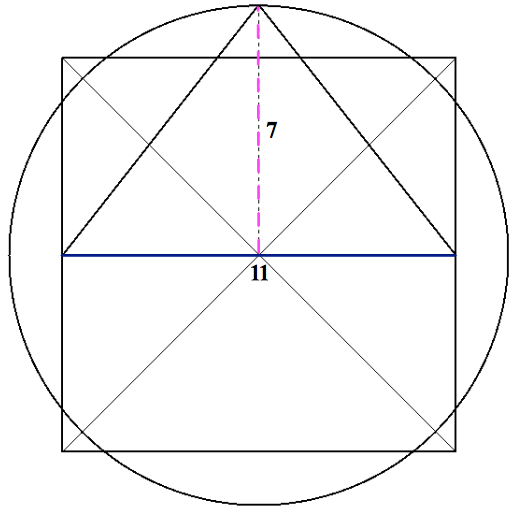(a) Squaring of the circle in the Great Pyramid (b) Rational approximation of π/2 in the height to base ratio of the Great Pyramid. Figure 3

Less known is the fact that the dimensions of the Great Pyramid also hide another important number in science, the Euler number e. Rick Howard's research has shown that the ratio of the two angles in the triangle a-b-h in the Great Pyramid (Table 1) provides a very accurate approximation er of e:

$β=tan-1(280220)=tan-1(1411)=51.842773413∘$

$er≡2β90-β=2,717323980=e±0.035%$

The following table illustrates and summarizes the three key numbers contained in the dimensions of Great Pyramid of Giza: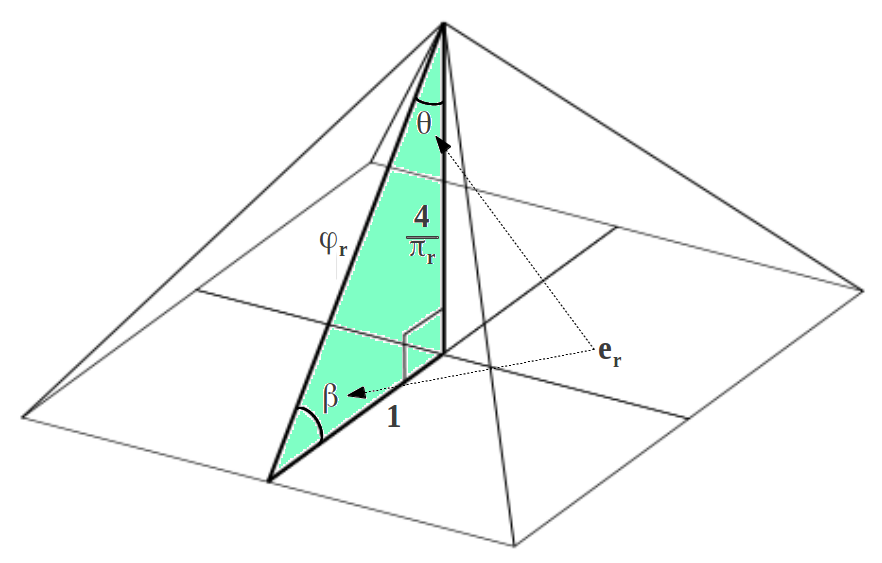Key Number Exact Value Great Pyramid Error φ 1.618033989 1.618590347 0.034% π 3.141592653 3.142857153 0.040% e 2.718281828 2.717323980 0.035% Table 2: Key numbers hidden in the dimensions of the Great Pyramid of Giza.

# 5.- The Great Pyramid contains the diameter of the Earth and Moon

If the preceding has not left the reader astonished, what follows probably will. The squaring of the circle in the Great Pyramid of Giza as shown in Figure 3a is a diagram that allows the precise determination of the diameter of both the Earth and the Moon. You "only" need to draw an incribed circle inside the square (the Earth) and a small circle centered at the apex and tangent with the preceding one (the Moon). At each side on top of them there are two exact 3-4-5 Pythagorean triangles (Figure 4).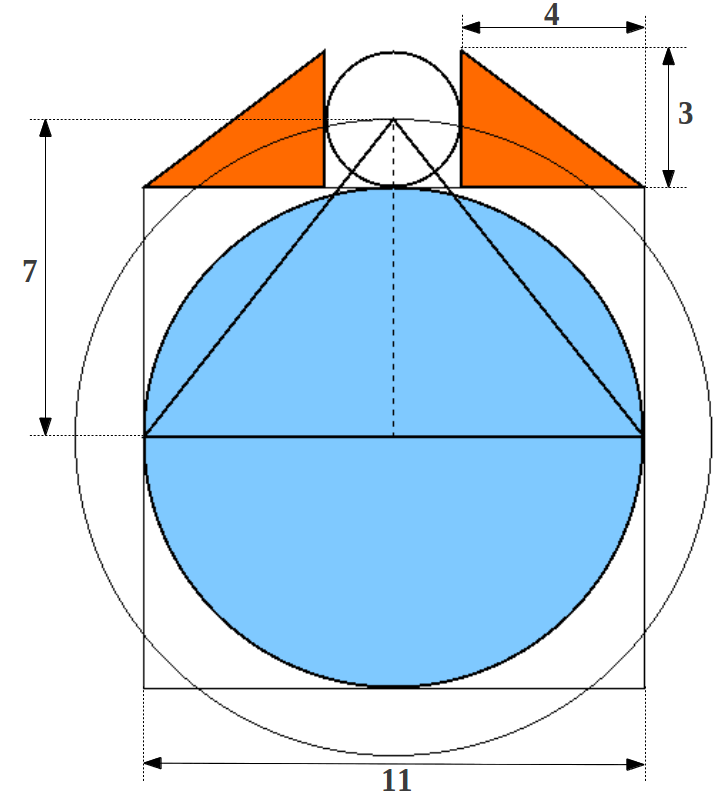Figure 4: The proportions in the Great Pyramid and the Phythagorean 3-4-5 triangle lead to the exact dimensions of the Earth and Moon (see also Figure 5).

This old known 3-4-5 triangle allows to calculate the "magic" number 720 as follows:

$3+4+5=123×4×5=60}⟶12×60=720$

From it and the proportions of the Great Pyramid as shown in Figure 4, the diameter of the Earth and Moon in miles can be directly determined!!!

$Earth:11×720=7920 milesMoon:3×720=2160 miles$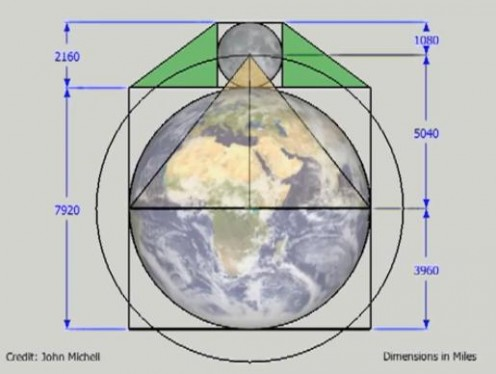# 6.- The architects of the Great Pyramid had a secret

Following this excellent article by Joseph Turbeville, in this section we're going to show how the exact dimensions of the Great Pyramid obey a very simple formula related to the cubes of some specific integer values. Those values can be easily obtained from a suitable arrangement of the first eight digits of our numeral system and their multiples, provided one knows the right units to use.

Consider Table 3a. Each row after the first one is formed by multiplying the first row times the row number. This way, the second row is twice the first one, the third one is three times the first one, and so on. The next step is to reduce each number to a single digit by consecutive summation, sometimes also called distillation (Table 3b). We have highlighted the key cells in Table 3b that will allow us to calculate the dimensions of the Great Pyramid.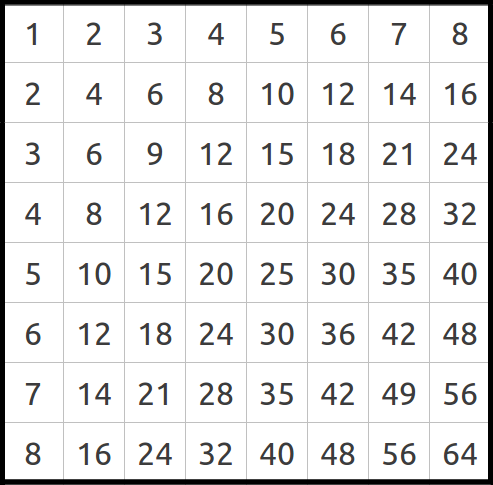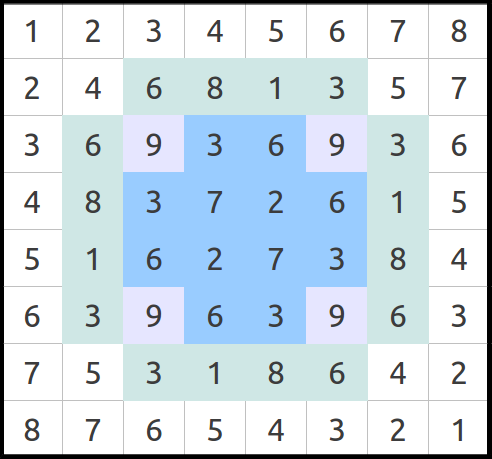(a) The first eight numbers and their multiples. Each row is the first one times the row number. (b) Reduction of the numbers in preceding table to a single digit. We have highlighted the numbers that allow to calculate the dimensions of the Great Pyramid. Table 3

Now the cubes of the four numbers in any of the surrounding lines (Table 4a) are added to obtain the first key number:

$63+83+13+33=756$

Do you recognize this number? Yes, using the feet as a unit, this is exactly the base length of the Great Pyramid.

$2b=756ft=440ec$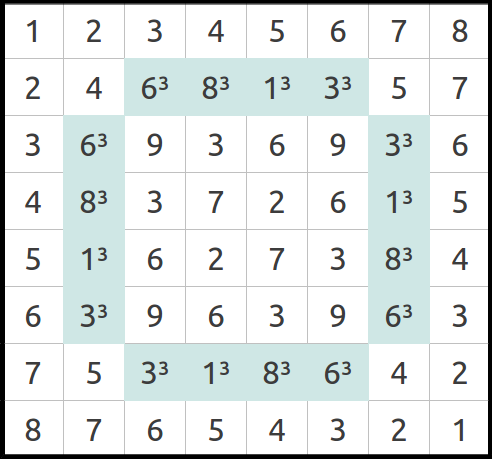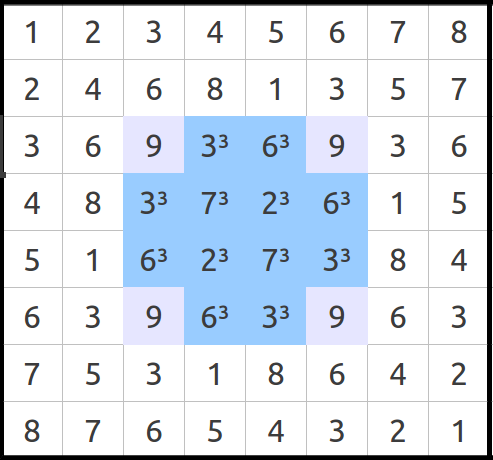(a) Adding the cubes of any of the highlighted lines provides the base length of the Great Pyramid in feet. (b) Adding the cubes of any line in the blue cross provides the exact ratio 4/πr. Table 4

Then any of the lines in the middle blue cross (Table 4b) provides the second key number, again after summation of the cubes:

$33+73+23+63=594$

If this number does not have any meaning for you, just notice where the quotient of both leads us:

$756594=1411=4πr$

As you can see, this is exactly the ratio that allows to calculate the height of the Great Pyramid given half its base:

$h=1411·378=481.1ft=280ec$

# 7. Conclusions

The Great Pyramid encodes much more information than the one summarized in this article. We encourage the reader to make his/her own search for information. At a higher astronomical scale, it is known that the Great Pyramid hides the grand cycle of Precession of the Equinoxes of our solar system around the central sun of the Pleyades (25827.5 years) in many of its dimensions (for example in the sum of the diagonals of its base expressed in pyramidal inches). It is also well known that the three pyramids in the Giza complex are aligned with the stars in the Belt of Orion. It appears that we can draw a single conclusion from all the preceding: the architects of the Great Pyramid of Giza were extremely wise beings, with an advanced knowledge of math and astronomy far beyond the standard of their time, and I would say even far beyond our own current knowledge.